Get instant live expert help with Excel or Google Sheets“My Excelchat expert helped me in less than 20 minutes, saving me what would have been 5 hours of work!”

#### Post your problem and you’ll get Expert help in seconds.

Your message must be at least 40 characters
Our professional Expert are available now. Your privacy is guaranteed.

# How to Use The Excel TIMEVALUE Function

In Excel, the time is stored as a serial number or decimal number, a number from 0 to 0.999988426 (12:00:00 AM to 11:59:59 PM). If the time is entered as text value then Excel does not recognize it and we can make calculations or manipulations using the text value of time. The Excel TIMEVALUE function converts the text representation of time into a decimal value of time.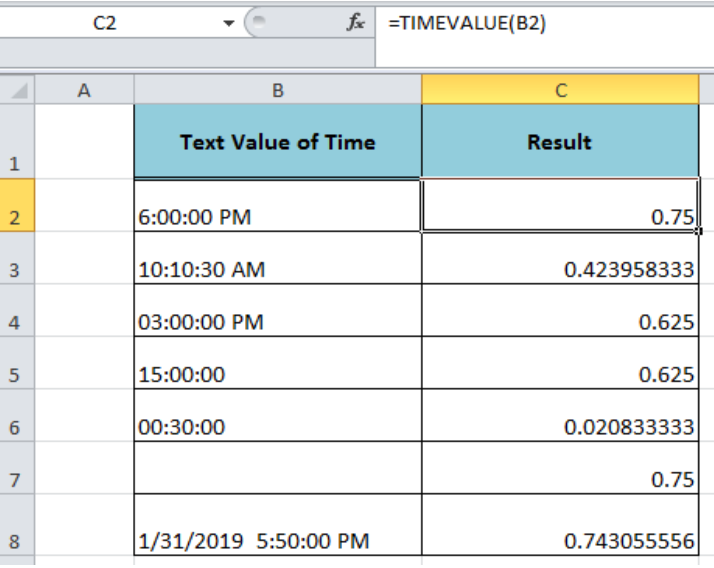Figure 1. Using the Excel TIMEVALUE Function

## Syntax

The syntax for the TIMEVALUE function is;

`=TIMEVALUE(time_text)`

Where,

• Time_text (required argument) – It is a text representation of time. We can enter this argument directly as a text string or cell reference that contains time value but formatted as text. The hours, minutes and seconds in the text string of time must be separated by colons.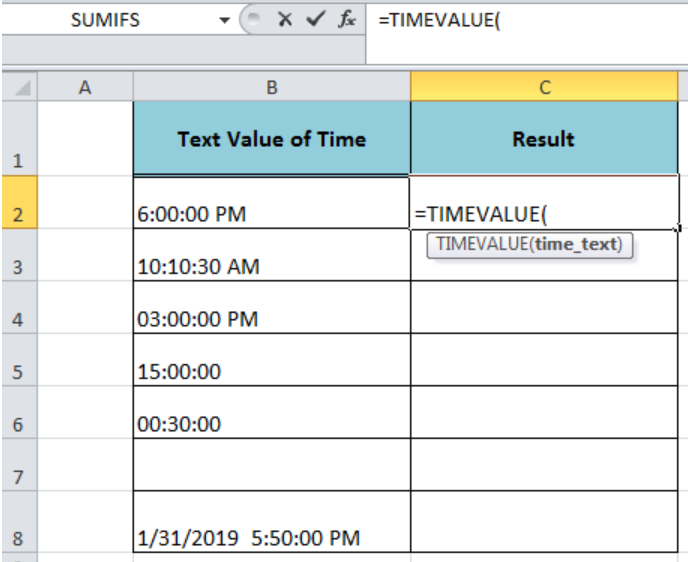Figure 2. The Syntax For the Excel TIMEVALUE Function

## Using the Cell Reference Formatted as Text

Let say we have text representation of time values in cell references of column B. Using the Excel TIMEVALUE we can convert these time values formatted as text into decimal values that are stored as time values in Excel, such as;

`=TIMEVALUE(B2)`

Copy this formula down to other cells to convert text representation of time into decimal values.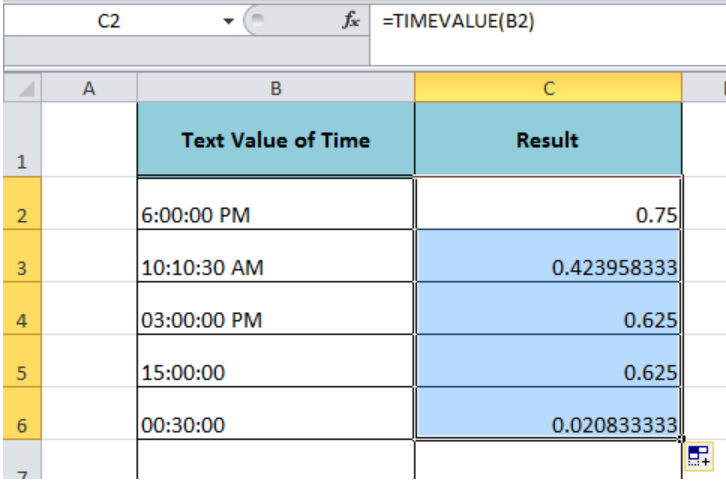Figure 3. Using the Cell Reference Formatted as Text

## Using Text String of Time Directly as Argument

We cant enter the text string of time directly in the time_text argument of the TIMEVALUE function to convert it as a decimal value of time, such as;

`=TIMEVALUE("6:00:00 PM")`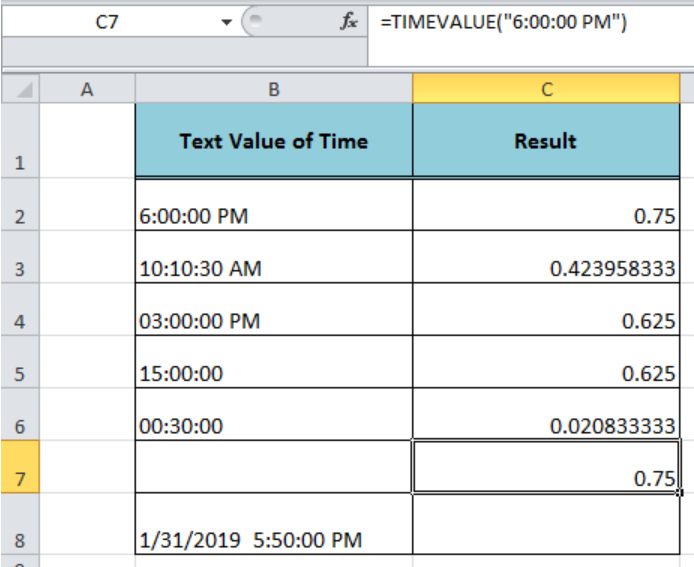Figure 4. Using Text String of Time Directly as Argument

## Using Date and Time value formatted as Text

If we have the date and time value in a cell that is formatted as text, we can easily convert the time part of this value into a decimal value of time by ignoring the date part of this value by using the TIMEVALUE function, such as;

`=TIMEVALUE(B8)  `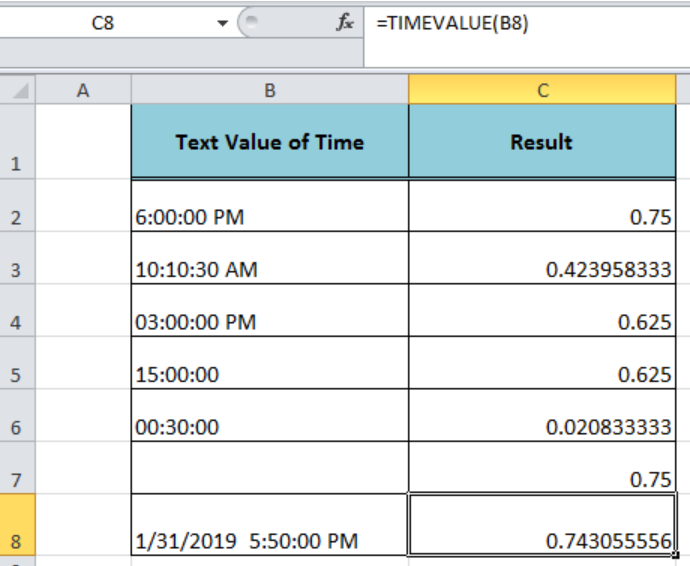Figure 5. Using Date and Time value formatted as Text

## Instant Connection to an Expert through our Excelchat Service:

Most of the time, the problem you will need to solve will be more complex than a simple application of a formula or function. If you want to save hours of research and frustration, try our live Excelchat service! Our Excel Experts are available 24/7 to answer any Excel question you may have. We guarantee a connection within 30 seconds and a customized solution within 20 minutes.

### Did this post not answer your question? Get a solution from connecting with the expert.Another blog reader asked this question today on Excelchat:## Subscribe to Excelchat.coAnother blog reader asked this question today on Excelchat: